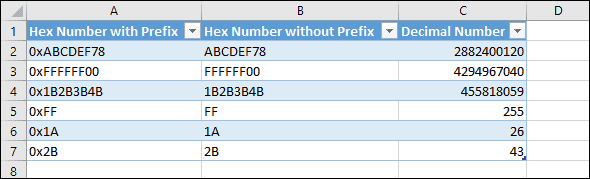# Working with HEX values in Excel

It’s unusual to need to work with Hexadecimal values in Excel, but on these rare occasions it can be challenging to figure out how Excel deals with values of this kind. If you simply enter a value like 0xABCDEF78 Excel will treat it as text and won’t recognize it as a numeric value.

Hexadecimal (or Hex for short) is a counting system that uses 16 symbols as opposed to the 10 symbols used in the Decimal counting system that we use more often. Hexadecimal uses the letters A-F as well as the numbers 0-9.

Hexadecimal is most often used by computer systems, though it is also used in some advanced mathematical calculations outside of the world of computing.

## Converting Hexadecimal values to Decimal in Excel

Excel won’t recognize a Hexadecimal value, but there is a function in its function library that will convert Hexadecimal values into Decimals: the HEX2DEC function.

For example: =HEX2DEC(“FF”) will return 255 – the decimal conversion of the Hexadecimal value FF.

If you’re unfamiliar with Excel functions and formulas you might benefit from our completely free Basic Skills E-book, which will introduce you to the basics of formulas and functions.

## Dealing with 0x prefixes

Many programming languages prefix Hexadecimal values with 0x. For example 0xFF instead of just FF.

The HEX2DEC function won’t recognize a Hex value that has a 0x prefix so you will need to strip away the prefixes before you can convert the values.

There are a few different ways you could do this, including Flash Fill, Text To Columns and even Find & Replace, but a formula offers the most long-term solution because it will automatically recalculate. This formula will remove the 0x prefixes:

=RIGHT(A2,LEN(A2)-2)

All of the options mentioned above (as well as the RIGHT and LEN functions) are explained in depth in our Expert Skills Books and E-books.

## A working example

You can download an example workbook showing the above functions and formulas in action.## Other counting systems supported by Excel

As well as the HEX2DEC function for Hex values, Excel also contains functions to convert values from Binary (Base 2) and Octal (Base 8). These are supported by the BIN2DEC and OCT2DEC.

There are also functions to convert each of these counting systems to the other. For example you can convert an Octal value into a Hex value using the OCT2HEX function.

Scroll to Top